## General Question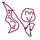# 3x = 81 how is this equation solved?

Asked by milla101 (221) July 15th, 2009

3x = 81 what is the value of “x”. The answer is 4…. but how in the WORLD do you get that answer? I thought it was 27.. =S

Observing members: 0Composing members: 0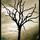because 3x here means “3 to the fourth power”, which would be 81 (3×3 x 3×3)

BBSDTfamily (6834)“Great Answer” (3) Flag as…Cheers, that makes perfect sense. =D

milla101 (221)“Great Answer” (0) Flag as…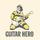but that is a kinda funk ass way of writing it. in basic algebra, the answer would be indeed 27.

Kiev749 (2092)“Great Answer” (1) Flag as…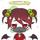@BBSDTfamily
i never heard of writing it that way.

ragingloli (50115)“Great Answer” (0) Flag as…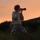Since when is ‘x’ to the fourth power? To me the answer is 27.

joeysefika (3093)“Great Answer” (0) Flag as…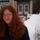The “x” needs to be in superscript for 4 to be the answer. Superscript means the “x” is floating at the top of the 3 in smaller text. Otherwise the answer is 27.

EmpressPixie (14755)“Great Answer” (0) Flag as…@EmpressPixie
ah of course.
but then he could have written it 3^x

ragingloli (50115)“Great Answer” (0) Flag as…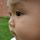Your teacher is testing you to see if you can get the right answer. lol

MrGV (4170)“Great Answer” (0) Flag as…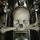Oops, I misread the question. Yes, it should have been written 3^x. If the teacher really wrote 3x=81, he/she must have made a typo.

b (1873)“Great Answer” (0) Flag as…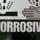@BBSDTfamily could please explain how you came to that conclusion of your answer? How could you have come up with “3 to the fourth power”? This equation might just be an inequality and it was purposly put in place by your teacher. You have to be able to prove your answer for it to be right.. I really don’t see how 3x means (3X3×3X3).

AtSeDaEsEpPoAoSnA (1502)“Great Answer” (0) Flag as…It has to be 27…

AtSeDaEsEpPoAoSnA (1502)“Great Answer” (0) Flag as…The answer of x = 4 implies that the problem should have been written as three to the power of 4.

b (1873)“Great Answer” (0) Flag as…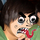the problem is 3x = 81. you have to find ‘x’

3x means ‘x’ is being multiplied by 3. you have the answer, 81, so you can use logic to determine that if 3 times ‘x’ is 81, then 81/3, or 81 divided by 3, equals ‘x’.

Icky (223)“Great Answer” (2) Flag as…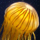answer is 4 i have been in the military for 5 years now and this question was apart of our first testing and answer is 4.

if you went for military and put 27 you would get a big fat X beside it.

LatinoNavySeal (7)“Great Answer” (1) Flag as…Response moderated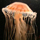Yeah this is a question on the first test for the defence force. I know cause i just did it lol. The answer is 4.

willo (1)“Great Answer” (0) Flag as…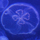We all know the answer is 4. But to UNDERSTAND WHY so you can hope to pass an exam with a question like this…. 3x =81 is 3 to the power of ? = 81, so we use 3 and times it buy itself how many times until we get 81.)This is how it works, 3×3x3×3 =81 or(3×3=9×3=27×3=81 YAY…:)

peter_pan (6)“Great Answer” (1) Flag as…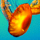-9

zulaika (1)“Great Answer” (0) Flag as…I have just come across this in the examples for the Defence Force Mathematical ability test. I answered 27, however was VERY sceptical as I had never seen the superscript like that, other than m2 square metres, m3 etc. The question (although I can’t type a superscript x so just imagine please) If 3x (superscript x) = 81, what is x. I looked and looked online. THE ANSWER I FOUND THAT MADE THE MOST SENSE TO ME WAS:

So we use 3 and times it buy itself how many times until we get 81.

as answered above by Peter Pan. THANK YOU PETER PAN

Hawker65 (6)“Great Answer” (0) Flag as…or